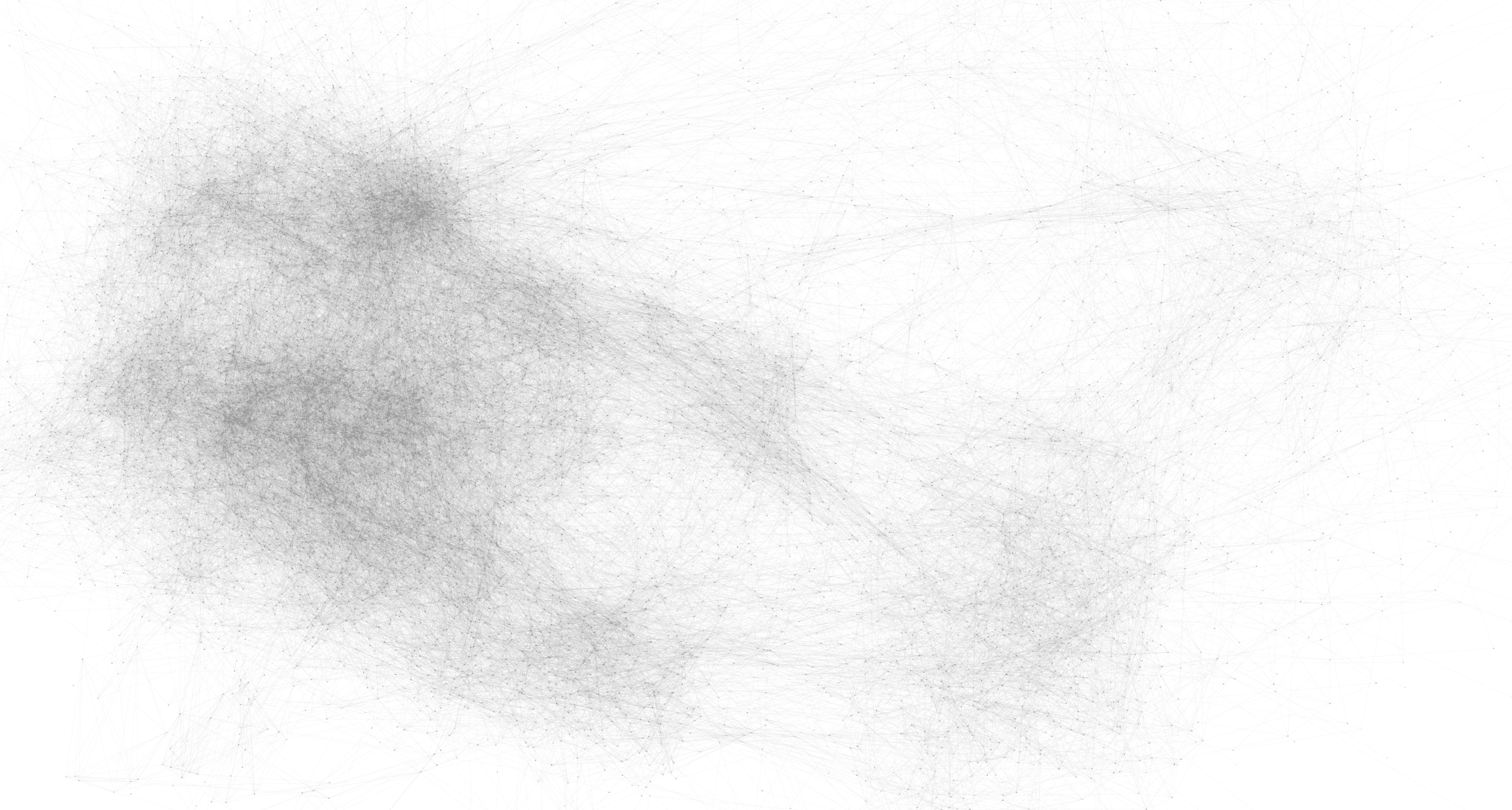# Writing a Weaver solver

Alexander Comerford
October 4th, 2022 · 2 min read

Almost every night my wife and I play Weaver together. It's always fun after several nights not finding the shortest "optimal" sequence of words it got me thinking. "How hard could it be to write an optimal Weaver solver?"

So I made one. Here's how I did it.

## Step 1: Getting the Weaver lexicon

Weaver is based around finding a sequence of 4 letter words that each differ by one letter and lead to some final word.

The obvious first step to making a Weaver solver is to get all English 4 letter words!

I started by downloading an English dictionary on GH and filtered out all non 4 letter words.

1wget https://github.com/dwyl/english-words/raw/master/words_alpha.txt -O /tmp/words.txt2awk '{ if (length($0) == 5 ) print }' /tmp/words.txt | uniq > /tmp/4words.txt3wc -l /tmp/4words.txt 17186 /tmp/4words.txt We see here that our lexicon is 7186 words. ## Step 2: Building the Weaver graph The next step is to build a representation of the Weaver "solution space" that we can work with to enable us to find the shortest word path. The simplest representation I could think of is a graph where the nodes are the words, and the edges link words that have a 1 letter difference. This representation is convenient because once we make it, we can run a shortest path graph algorithm (like Djikstra's) to get the optimal word path. 1def is_distance_one(word1, word2):2 """If the words share 3 letters, they are distsance one"""3 word1 = set((i, w) for i, w in enumerate(word1))4 word2 = set((i, w) for i, w in enumerate(word2))5 return len(set(word1).intersection(set(word2))) == 36 7weaver_graph = {}8with open('/tmp/4words.txt', 'r') as four_words:9 for new_word in map(lambda w:w.strip(),four_words):10 weaver_graph[new_word] = []11 for existing_word, possible_next_words in weaver_graph.items():12 if is_distance_one(new_word, existing_word):13 weaver_graph[new_word].append(existing_word)14 weaver_graph[existing_word].append(new_word)15 16import json17with open("/tmp/weaver_graph.json","w+") as f:18 f.write(json.dumps(weaver_graph)) This is a naive algorithm to create the graph, but it's simple, to the point, and we only have to run it once. Now that the graph is built, let's try visualizing it!Full view of the Weaver word graph This picture doesn't tell us much besides dense vs. not dense, but it's a pretty picture nonetheless! If you want explore the graph for yourself, here is the JSON blob and here is the SVG viz. ## Step 3: Building the Weaver solver! Now that we have a graph of all the Weaver words and the transitions between them, all we have left to do is build a solver! As mentioned we can now use a Djikstra's shortest path algorithm to find our optimal solution! 1import json2import heapq3from collections import namedtuple4from dataclasses import dataclass5 6@dataclass7class QueueItem:8 node: str9 path: list[str]10 cost: int = 111 12 def __lt__(self, other):13 return self.cost < other.cost14 15class WeaverSolver:16 def __init__(self, weaver_graph_path: str):17 self.weaver_graph = json.loads(open(weaver_graph_path,"r").read())18 19 def solve(self, start_word: str, end_word: str, avoid_words: list[str] = None) -> list[str]:20 assert start_word in self.weaver_graph, f"Start word {start_word} not in graph"21 assert end_word in self.weaver_graph, f"End word {end_word} not in graph"22 23 if not avoid_words:24 avoid_words = []25 26 init_queue_item = QueueItem(node=start_word, path=[], cost=0)27 queue = [init_queue_item]28 seen = set()29 30 while True:31 qi = heapq.heappop(queue)32 if qi.node not in seen:33 34 qi.path = qi.path + [qi.node]35 seen.add(qi.node)36 37 # if we are at the end, return the path38 if qi.node == end_word:39 return qi.path40 41 # search edges avoiding nodes and increasing cost42 for search_node in self.weaver_graph[qi.node]:43 cost = float("inf") if search_node in avoid_words else qi.cost + 144 heapq.heappush(45 queue,46 QueueItem(47 node=search_node,48 path=qi.path,49 cost=cost,50 )51 )52 53solver = WeaverSolver("/tmp/weaver_graph.json")54print(solver.solve("bone", "cast")) 1['bone', 'bane', 'cane', 'cant', 'cast'] And huzzah! We have a Weaver solver! ## [UPDATE] Step 4: Word frequency weights One problem I noticed with this solver is that sometimes the word path chosen won't get accepted by Weaver. To try and remedy this without Weaver's word list I decided to try and "weight" the graph edges based on word frequency. First I got a frequency list of english words by usage 1wget https://github.com/nachocab/words-by-frequency/raw/master/english.txt -O /tmp/words_freq.txt2awk '{if(length($2)==4){print $2,$1}}' /tmp/words_freq.txt | tr " " "," > /tmp/4words_freq.csv3cat /tmp/4words_freq.csv | python3 -c 'import json,sys,pandas;print(json.dumps(pandas.read_csv(sys.stdin,header=None).set_index(0).to_dict()))' | jq -r > /tmp/4words_freq.json

Then I adjusted the code we had before except added a "likeness" factor to make edges whose words are more popular slightly less expensive to traverse.

While it adds a hyperparamater to tune how "closely" we follow this frequency list, it makes us much more likely to get a path accepted by Weaver.

1import json2import heapq3from collections import namedtuple4from dataclasses import dataclass5
6@dataclass7class QueueItem:8    node: str9    path: list[str]10    cost: int = 111
12    def __lt__(self, other):13        return self.cost < other.cost14
15class WeaverSolver:16    def __init__(self, weaver_graph_path: str, word_freq: str = "", freq_likeness: int = 5):17        self.weaver_graph = json.loads(open(weaver_graph_path,"r").read())18
24        self.freq_likeness = freq_likeness25
26    def solve(self, start_word: str, end_word: str, avoid_words: list[str] = None) -> list[str]:27        assert start_word in self.weaver_graph, f"Start word {start_word} not in graph"28        assert end_word in self.weaver_graph, f"End word {end_word} not in graph"29
30        if not avoid_words:31            avoid_words = []32
33        init_queue_item = QueueItem(node=start_word, path=[], cost=0)34        queue = [init_queue_item]35        seen = set()36
37        while True:38            qi = heapq.heappop(queue)39            if qi.node not in seen:40
41                qi.path = qi.path + [qi.node]42                seen.add(qi.node)43
44                # if we are at the end, return the path45                if qi.node == end_word:46                    return qi.path47
48                # search edges avoiding nodes and increasing cost49                for search_node in self.weaver_graph[qi.node]:50                    cost = 151                    if search_node in avoid_words:52                        cost = float("inf")53                    elif search_node in self.word_freq:54                        cost = qi.cost + 1 - (self.word_freq[search_node] / (self.freq_likeness*self.word_freq[search_node]+1))55                    else:56                        cost = qi.cost + 157                    heapq.heappush(58                        queue,59                        QueueItem(60                            node=search_node,61                            path=qi.path,62                            cost=cost,63                        )64                    )65
66solver = WeaverSolver(weaver_graph_path="/tmp/weaver_graph.json", word_freq="/tmp/4words_freq.json", freq_likeness=5)67print(solver.solve("snow", "days"))
1['snow', 'snot', 'soot', 'boot', 'boos', 'boys', 'bays', 'days']